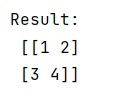# How to Convert a Tensor to NumPy array in Tensorflow?

In this tutorial, we will learn how to convert a tensor to NumPy array in tensorflow in Python? By Pranit Sharma Last updated : May 24, 2023

## Importing Transferflow

To import the transferflow, follow the below given statement:

```import tensorflow as tf
```

## Creating Tensor Object

To create an object of tensor, just use the tensorflow.constant() method. Consider the following statement:

```tf.constant([[1, 2], [3, 4]])
```

## Converting a Tensor to NumPy Array in Tensorflow

To convert the tensor to a NumPy array, you can use t.numpy() method, where t is an object of tensorflow.

Let us understand with the help of an example,

## Python Program to Convert a Tensor to NumPy array in Tensorflow

```# Import numpy
import numpy as np

# Import tensorflow
import tensorflow as tf

# Creating a tensor object
t = tf.constant([[1, 2], [3, 4]])

# Converting into numpy object
res = t.numpy()

# Display result
print("Result:\n",res)
```

### Output